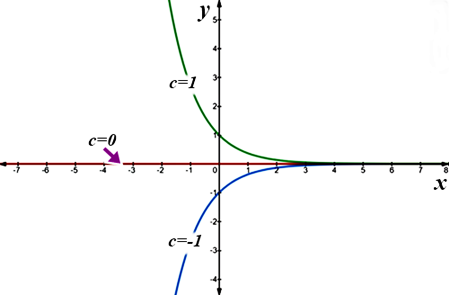# Let f(x,y) = ye^x. Draw the level curves f(x,y) = c for c = -1, 0, 1.

## Question:

Let {eq}f(x,y) = ye^x {/eq}. Draw the level curves f(x,y) = c for c = -1, 0, 1.

## Level Curves:

The surface graphics of the {eq}z = f\left( {x,y} \right) {/eq} functions are usually a bit difficult. It is common for these functions to use level curves called contour lines. If a function is given by the expression {eq}z = f\left( {x,y} \right) {/eq} and we do {eq}f(x,y) = c {/eq}, this equation corresponds to the surface points obtained by sectioning with the plane {eq}z=c {/eq}, parallel to the plane of coordinates {eq}z=0 {/eq}. For different {eq}c {/eq} values, different contours form a family of contour lines.

{eq}\eqalign{ & {\text{We can say that a level curve is the set of points in the plane where the function }}z = f\left( {x,y} \right){\text{ }} \cr & {\text{has a constant value }}\,f(x,y) = c{\text{.}} \cr & {\text{Given the function }}\,f\left( {x,y} \right) = z = y{e^x}\,\,{\text{ its contours are given by:}} \cr & \,\,\,\,\,y{e^x}\, = c \cr & {\text{So by placing values to }}\,c{\text{, we get a family of exponential curves in the }}xy{\text{ - plane:}} \cr & {\text{ }}\,c = - 1\,\, \Rightarrow y{e^x} = - 1\,\,\, \Rightarrow y = - {e^{ - x}} \cr & {\text{ }}\,c = 0\,\, \Rightarrow y{e^x} = 0\,\,\, \Rightarrow y = 0 \cr & {\text{ }}\,c = 1\,\, \Rightarrow y{e^x} = 1\,\,\, \Rightarrow y = {e^{ - x}} \cr} {/eq}